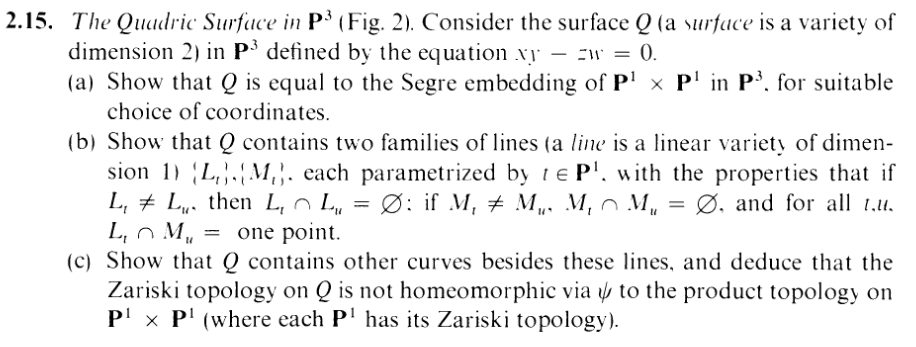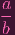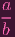I.2.15c

3/9/2021This exercise has, let's say, a twisted ending. First of all, we are asked to find curve(s) other than the lines I found back in
part (b) last time. So to show that P1 × P1 and Q aren't homeomorphic under ψ, the implicit suggestion is that we have to show that closed sets in the former map only to lines in the latter. Hence, ψ will "miss" the special nonline curve(s) in Q.

Lemme save finding such a "nonline" curve for later, and for now let me show that ψ does indeed only map closed sets to lines.

I struggled quite a bit with this, for the same reason I struggled quite a bit all the way back in 1.4: The product topology is oddly weird to think about.

Now what does an arbitrary open set in the product topology of P1 × P1 look like?

An arbitrary open set looks like iUi ×V i, where Ui and V i are open in P1. Now, let me define the closed sets Zi = P1 - U and Ci = P1 - V .

Then an arbitrary closed set in P1 × P1 can be written as

 P1 × P1 -⋃ iUi × V i = ⋂ iP1 × P1 - (Ui × V i) = ⋂ i((P1 - Ui) × P1) ∪ (P1 × (P1 - V i)) (See here) = ⋂ i(Zi × P1) ∪ (P1 × Ci)

Now, my friends, let's take a look at, say Zi × P1. Reader: I'm going to have to have you trust me on the following: The closed sets in P1 are all either finite, empty, or just P1 itself (similar to A1). If Zi is empty, that's a degenerate case (then the whole set is empty so the image under ψ is also empty). If Zi = P1, then that's also a trivial case (the image under ψ is just the entirety of Q). So the only nontrivial case is Zi being finite.

Basically, Z1 × P1 can be rewritten as the union of finitely many sets of the form {PP1, where {P} is a singleton. So let's actually restrict our attention to just {PP1, where P = (a,b)

Now what does ψ do to an arbitrary element (a,b,c,d) ∈{PP1? It sends it to (W,X,Y,Z) = (ab,ac,bc,bd). But keep in mind that a and b are constants in our scenario (well, up to a constant multiple lol), since they are the coordinates of P. So our point satisfies

 W =Y X =Z

(on the constant multiple thing: note that if I used (ka,kb) instead, te ks cancel out)

Look familiar? Maybe not, because now I'm using W,X,Y,Z instead of A,B,C,D (because I realize that those are a more appropriate choice of coordinate names if our coordinate ring is k[w,x,y,z], lol). But if you look closely, this is just what I called a "TYPE K" line. Muahahaha. So our {PP1 is just getting mapped to a TYPE K line (So Zi × P1 is just getting mapped to a union of TYPE K lines). Or, to be more precise I showed that it's getting mapped to a subset of a TYPE K line, but it's easy to show that the reverse inclusion holds as well (I won't show it here)

"But Mr. Holeinmyproof, you just divided by b! What if b = 0? What then?" Good question, but don't call me that again. What does happen if b = 0? Well, then our image point would instead be (W,X,Y,Z) = (ab,ac,bc,bd) = (ab,ac, 0, 0). I.e. we'd get

 Y = 0 Z = 0

Look familiar? Again, I'm using W,X,Y,Z now instead, but this is just what I called a
TYPE H line. Remember how types H and K ended up going together, as what I called "TYPE M" lines?

So, basically, Zi × P1 gets mapped to a union of TYPE H and K lines (union of "TYPE M" lines).

Similarly, you can guess that P1 × Ci gets mapped to a union of TYPE I and J lines (union of "TYPE L" lines).

So in total, our arbitrary closed set gets mapped to intersections of lines in Q, which we showed back in part (b) are either empty, singletons, or lines themselves.

So ψ maps closed sets only to lines in Q (or more trivial things: singletons, empty sets, P1 × P1 itself). DONE WITH THAT PART.

Now all I have to show is that there are in fact nonline curves in Q, and I'm done

Okay.

*Sigh*

*Sigh*

So, remember how in Club Penguin you used to dress up as a pink Penguin and *Sigh* in the corner of the pizza place so that other Penguins would come to you and ask you "what's wrong?" and you could get easy attention and pity that way, as well as possibly an e-bf with whom you could go on romantic dates and have e-sex with in your igloo?

What? Just me? Okay, well, anyway, that's how I feel right now. I feel like a lonely, sighing pink penguin in the corner.

The reason is.... Early on, in yesterday's post, I had a little mini freak out about how our xy - zw looks super familiar to one of the polynomials used to generated [CENSORED]? Well, in particular, it looks familiar to the xw - yz polynomial you can find on the Wikipedia page.

Plot twist: They're actually the fucking same. The reason is that the Wikipedia page (as well as my work in 2.12d) uses the coordinate ring k[x,y,z,w], whereas here we're using the coordinate ring k[w,x,y,z]. If you just relabel the coordinates, you'll see that they're actually the same surface. Yes, the quadric surface Q actually contains the t-t-t-tWISTED CUBIC CURVE.

SO, YOU WANT A NONLINE CURVE IN Q, MOTHERFUCKERS? YOU WANT IT? WELL, I'M GOING TO USE THE TWISTED MOTHERFUCKING CUBIC CURVE AS MY EXAMPLE (SINCE THE TWISTED CUBIC CURVE IS OBVIOUSLY NOT A LINE, ESPECIALLY NOT ONE OF THE TYPE M OR TYPE L LINES FROM Q), AND THIS EXERCISE IS FINISHED.

Yes, the twisted cubic curve actually saved me. Maybe it doesn't hate me after all?

Would it ask me *what's wrong* if I sighed in the corner?